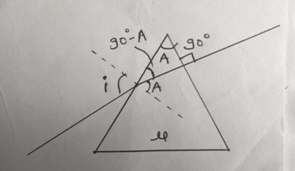Question

# A ray is incident at an angle of incidence on one surface of a prism of small angle and emerges normally from the opposite surface. If the refractive index of the material of the prism is , the angle of incidence is nearly equal to :

A
B
C
D
Medium
Updated on : 2022-09-05
SolutionVerified by Toppr
Correct option is D)

## Hint: Here, we have to apply the concept of angle of deviationSolution:Step1: Draw free body diagramStep2: Apply given conditions According to free body diagram,i=Angle of incident, A=Small angle of prism & µ=Refractive index of material Assume, e= angle of emergence given that, the incident ray emerges normally from the opposite surface,Hence, Step3: Find angle of deviationWe know the angle of deviation () can be, In case of prism, we know that, sum of small angle of prism & angle of deviation in a prism is equal to the sum of angle of incidence and angle of emergence. So, we get, Submit values from equation (1) & (2) in equation (3) we get, or Hence, option (D) is correct.

Video Explanation00